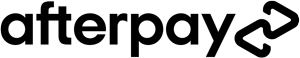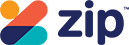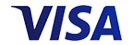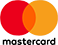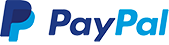Grid List
WAS \$39.95 NOW \$29.95
Increase value Decrease valueWAS \$24.95 NOW \$19.95
Increase value Decrease value
WAS \$12.95 NOW \$9.95
Increase value Decrease value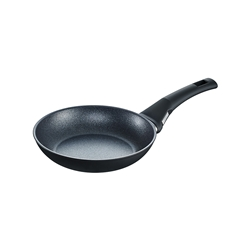WAS \$89.95 NOW \$49.95
WAS \$12.95 NOW \$8.95WAS \$49.95 NOW \$39.95
Increase value Decrease value
WAS \$9.95 NOW \$4.99
Increase value Decrease value
WAS \$12.95 NOW \$9.95
Increase value Decrease value
WAS \$12.95 NOW \$9.95
Increase value Decrease valueWAS \$24.95 NOW \$19.95
Increase value Decrease value
WAS \$12.95 NOW \$9.95
Increase value Decrease value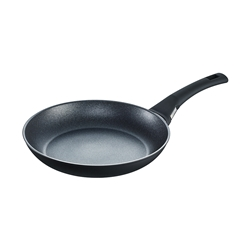WAS \$119.95 NOW \$69.95
Increase value Decrease value